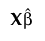Publication date: 08/13/2020

##Actual by Predicted Plot

The Actual by Predicted plot appears by default. It provides a visual assessment of model fit that reflects variation due to random effects. It plots the observed values of Y against the marginal predicted values of Y. These are the predicted values obtained if you select Save Columns > Prediction Formula.

Denote the linear mixed model by E[Y|γ] = Xβ + Zγ. Here β is the vector of fixed effect coefficients and γ is the vector of random effect coefficients. The marginal predictions are the predictions from the fixed effects part of the predictive model, given by.